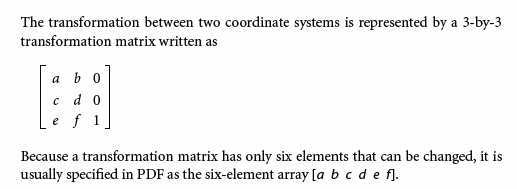# How do you find the resolution of a rotated image within a pdf?

Hello Tim,

It would be great to know what your overall objective is. Why is getting the resolution important for you?

Once we have a better idea of what you are trying to accomplish, then we can advise on the best solution.

Regards,

Ryan Barr

Software Developer | PDFTron Systems, Inc.

Ryan,

I work for a large book manufacturer, and my current project is to write some vb code for some basic preflight procedures on pdf’s using PDFTron. Some of these procedures include - finding page trim size, find any missing fonts, find any text that uses a missing font and highlight it, adding trim lines, find all used colors, search for specific text, find and highlight any element that is too close to the page edge (page bleed warning), and find/highlight any low-res image. (some are for customer reference/use, and some are for prepress operator use) I’m stuck on finding the resolution on rotated images within the pdf as I’m getting inaccurate results. I’ve tried finding the average scaling x,y for the image, and then calculating dpi (as suggested on this forum) with inaccurate results. I’ve also tried removing the rotation angle from the image and then calculating the dpi without writing the modified image with no success. The CTM is a little bit tricky to understand, so I’ve spent a lot of time reading through this forum, reading the PDF Reference, and writing and re-writing vb code. Any guidance would be greatly appreciated.

Have you tried to decompose the CTM? This forum post shows how to do so.
https://stackoverflow.com/questions/45159314/decompose-2d-transformation-matrix

From the PDF standard.So matching the SO post and PDFNet SDK you have
Xx : m_a
Xy : m_b
Yx : m_c
Yy : m_d

We don’t need to consider m_h or m_v because that is just displacement.

That will give you your scales, and then taking PDF at 72 DPI, and getting the Image height and width, I believe you can get your effective resolution.

I did not get a chance to test but I think the following is what will work.

`Matrix2D ctm = element.GetCTM(); double scalex = Math.Sqrt((ctm.m_a * ctm.m_a) + (ctm.m_b * ctm.m_b)); double scaley = Math.Sqrt((ctm.m_c * ctm.m_c) + (ctm.m_d * ctm.m_d)); double rot_angle = Math.Atan2(ctm.m_b, ctm.m_c);`

Thanks Ryan. I’m now testing with the following vb code which seems to work on all images except for ones with shearing (dpi values are off). Any direction on this?

'determine effective resolution of image

Dim imagewidth As Double = element.GetImageWidth()

Dim imageheight As Double = element.GetImageHeight()

Dim mtx As Matrix2D = element.GetCTM()

Dim scalex As Double = Math.Sqrt((mtx.m_a * mtx.m_a) + (mtx.m_b * mtx.m_b))

Dim scaley As Double = Math.Sqrt((mtx.m_c * mtx.m_c) + (mtx.m_d * mtx.m_d))

Dim xRes As Double = Math.Abs(imagewidth * 72 / scalex)

Dim yRes As Double = Math.Abs(imageheight * 72 / scaley)

'determine rotation angle of image

Dim radians As Double = Math.Atan2(mtx.m_b, mtx.m_a)

Dim rot_angle As Double = Int(radians * (180 / Math.PI))

Would it be possible to send me a PDF file with a sheared image, and also let me know what effective resolution you expect the sheared image to be.

If you can not post here, please send to support.

Here is a single page pdf with a single skewed image that has a resolution of 72 x 72 dpi. After running my code above, I’m getting 72 x 67 dpi.

Untitled-1.pdf (31.9 KB)

The following C# works in my tests, and for this file. This returns 72x72 for your skewed image for example.

`
static Tuple<bool, double, double> GetEffectiveResolution(Element element)
{
Matrix2D ctm = element.GetCTM();

double len = Math.Sqrt(ctm.m_a * ctm.m_a + ctm.m_b * ctm.m_b);
if(len == 0.0)
{
return new Tuple<bool, double, double>(false, -1.0, -1.0);
}

Matrix2D sc = new Matrix2D(1, 0, 0, 1, 0, 0);
sc.m_a = len;
sc.m_d = (ctm.m_a * ctm.m_d - ctm.m_b * ctm.m_c) / len;

double scalex = sc.m_a;
double scaley = sc.m_d;
int img_w = element.GetImageWidth();
int img_h = element.GetImageHeight();
double eff_res_x = img_w / scalex * 72.0;
double eff_res_y = img_h / scaley * 72.0;

return new Tuple<bool, double, double>(true, eff_res_x, eff_res_y);
}
`

Usage would be

`static void ImageExtract(PDFDoc doc, ElementReader reader) { Element element; while ((element = reader.Next()) != null) { switch (element.GetType()) { case Element.Type.e_image: case Element.Type.e_inline_image: { var eff_res_result = GetEffectiveResolution(element); if(eff_res_result.Item1) { Console.WriteLine(String.Format("Image : {0} {1}", eff_res_result.Item2, eff_res_result.Item3)); } break; } case Element.Type.e_form: // Process form XObjects { reader.FormBegin(); ImageExtract(doc, reader); reader.End(); break; } } } }`